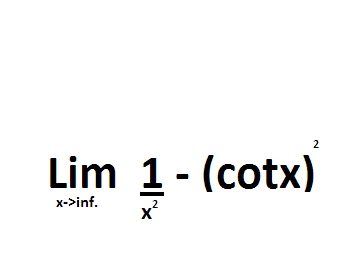# L'hospital's Rule Problem

Using L'hospitals rule, find the limitI seem to stuck using L'hospital's rule ,I replaced cotx=1/tanx but the derivatives of even 4th order are not simplying things.

Dick
Homework Helper
Before you even start with l'Hopital's rule you need to check you can write the limit in an indeterminant form 0/0 or infinity/infinity. Did you check that? And do you really mean lim x->inf??

Last edited:
Before you even start with l'Hopital's rule you need to check you can write the limit in an indeterminant form 0/0 or infinity/infinity. Did you check that?
Yes,
put cotx=(tanx)^-1, we have then
tan^2x-x^2
--------------
x^2tan^2x
Then we can use the rule.

But I tried wolfram alpha just now and found out that this limit does not exist.
http://www.wolframalpha.com/input/?i=limit+x%5E%28-2%29-%28cotx%29%5E2%2C++x-%3Einf

http://www.wolframalpha.com/input/?i=limit+x%5E%28-2%29-%28cotx%29%5E2%2C++x-%3E0
This is the same answer given in textbook. Probably a printing error.
Sorry If I wasted your time.

Dick
Homework Helper
Yes,
put cotx=(tanx)^-1, we have then
tan^2x-x^2
--------------
x^2tan^2x
Then we can use the rule.

But I tried wolfram alpha just now and found out that this limit does not exist.
http://www.wolframalpha.com/input/?i=limit+x%5E%28-2%29-%28cotx%29%5E2%2C++x-%3Einf